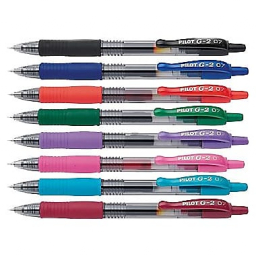# The cost 7

The cost of a pen is Rs. 20/3, and that of a pencil is 25/6. Which costs more and by how much?

Result

x = (Correct answer is: pen)d =  2.5 Rs

### Step-by-step explanation:Did you find an error or inaccuracy? Feel free to write us. Thank you!

Tips for related online calculators
Need help calculating sum, simplifying, or multiplying fractions? Try our fraction calculator.